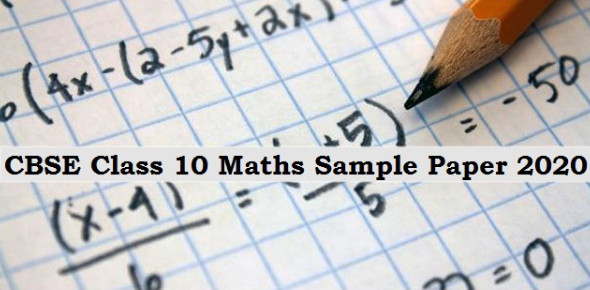# CBSE 10th Maths Quiz

124 Questions | Attempts: 2157
ShareSettingsUnlimited attempts possible. Overall 500+ questions available for practice. On each attempt, you will get 30 questions randomly.

• 1.
Find the [HCF X LCM] for the numbers 100 and 190.
• 2.
If the diameter of a semi-circular protractor is 14  cm, then find its perimeter
• 3.
If sec2θ (1 + sin θ)(1 – sin θ) = k, then find the value of k.
• 4.
Which measure of central tendency is given by the x-coordinate of the point of intersection of the "more than ogive " and "less than ogive” ?
• 5.
From a well shuffled pack of cards, a card is drawn at random.  Find the probability of getting a balck queen
• 6.
Which term of the progression 4,9,14,…. Is 109 ?
• A.

12

• B.

18

• C.

20

• D.

22

• 7.
If 1+2+….+k=55, then the value of k is
• A.

10

• B.

11

• C.

12

• D.

13

• 8.
The 10th term of the sequence whose nth term is (3n-2) is
• A.

28

• B.

42

• C.

35

• D.

32

• 9.
If cot A = 12/5, then the value of (sin A + cos A) x cosec A is :
• A.

13/5

• B.

17/5

• C.

14/5

• D.

1

• 10.
Given that sin A = 1/2  and cos B= 1/ then the value of (A+B) is :
• A.

30 Degree

• B.

45 Degree

• C.

75 Degree

• D.

15 Degree

• 11.
When a point is observed, the angle formed by the line of sight with the horizontal level where the point being viewed is above the horizontal plane is known as:
• A.

Angle of triangle

• B.

Angle of depression

• C.

Angle of elevation

• D.

None of the these

• 12.
The HCF of two numbers a and b is 30, while their LCM is 45. What is the value of (a x b) ?
• A.

1250

• B.

1255

• C.

1350

• D.

1355

• 13.
Solve for x :   2x + y = 8,    y= - 6
• A.

6

• B.

4

• C.

7

• D.

16

• 14.
Probability of an event can be  -1/6
• A.

True

• B.

False

• 15.
In a lottery there are 10 prizes and 20 blank. What is the probability of getting prize?
• A.

1/2

• B.

1

• C.

1/3

• D.

1/10

• 16.
The sum of exponents of prime factors of number 72072 is divisible by _
• A.

3

• B.

4

• C.

5

• D.

6

• 17.
The quadratic polynomial whose sum and product of zeroes are -3 and 4 respectively, among the following is _
• A.

- 3x + 4

• B.

- 3x -4

• C.

+ 3x -4

• D.

+ 3x +4

• 18.
The point of intersection of the lines 2x - y = 6 and x + 2y = -2 is
• A.

(2, -2)

• B.

(-2, -2)

• C.

(-2 , 2)

• D.

(2, 2)

• 19.
If the corresponding sides of two similar triangles are in the ratio 16:9, then the ratio of thier areas is
• A.

16:9

• B.

9:16

• C.

256:81

• D.

81:256

• 20.
If the median and mode of an ungrouped data are 25.6 and 21.4 respectively, then the mean of the data is
• A.

27.4

• B.

27.5

• C.

27.6

• D.

27.7

• 21.
If tan = cot  , then  =
• A.

30

• B.

45

• C.

60

• D.

90

• 22.
X = 2 cosec, y = 2 cot then -=
• A.

2

• B.

-2

• C.

4

• D.

-4

• 23.
+ =
• A.

.

• B.

-

• C.

1

• D.
• 24.
If the HCF of 612 and 1314 is 18, then thier LCM is
• A.

44676

• B.

44667

• C.

46674

• D.

46476

• 25.
The  form 0f 0. is
• A.
• B.
• C.
• D.

## Related TopicsBack to top
×

Wait!
Here's an interesting quiz for you.